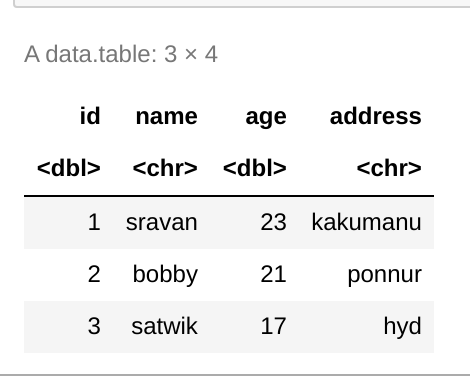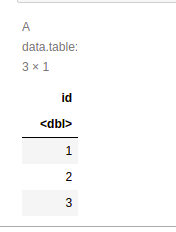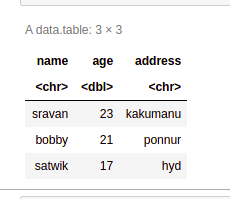# Remove Multiple Columns from data.table in R

• Last Updated : 31 Aug, 2021

In this article, we are going to see how to remove multiple columns from data.table in the R Programming language.

Create data.table for demonstration:

## R

 `# load the data.table package``library``(``"data.table"``)`` ` `# create a data.table with 4 columns``# they are id,name,age and address`` ` `data = ``data.table``(id = ``c``(1,2,3) ,``                  ``name = ``c``(``"sravan"``,``"bobby"``,``"satwik"``),``                  ``age = ``c``(23,21,17),``                  ``address = ``c``(``"kakumanu"``,``"ponnur"``,``"hyd"``))`` ` `# display``data`

Output:Here we are going to remove multiple columns by using the index operator []

Syntax: data[ ,’:='(column1 = NULL, column2 = NULL, column n=NULL)]

where

• data is the input data.table
• column is the columns to be removed
• := is the operator to be loaded in the data.table

Example 1: R program to remove multiple columns from data.table

## R

 `# load the data.table package``library``(``"data.table"``)`` ` `# create a data.table with 4 columns``# they are id,name,age and address`` ` `data = ``data.table``(id = ``c``(1,2,3),``                  ``name = ``c``(``"sravan"``,``"bobby"``,``"satwik"``),``                  ``age = ``c``(23,21,17),``                  ``address = ``c``(``"kakumanu"``,``"ponnur"``,``"hyd"``))`` ` `# remove age , name and address columns``data[ ,``':='``(age = ``NULL``, address = ``NULL``, name=``NULL``)]  `` ` `# display``data`

Output:Example 2: Remove only one column from data.table

## R

 `# load the data.table package``library``(``"data.table"``)`` ` `# create a data.table with 4 columns``# they are id,name,age and address`` ` `data = ``data.table``(id = ``c``(1,2,3),``                  ``name = ``c``(``"sravan"``,``"bobby"``,``"satwik"``),``                  ``age = ``c``(23,21,17),``                  ``address = ``c``(``"kakumanu"``,``"ponnur"``,``"hyd"``))`` ` `# remove id column``data[ ,``':='``(id=``NULL``)]  `` ` `# display``data`

Output:Example 3: R program to remove all columns

## R

 `# load the data.table package``library``(``"data.table"``)`` ` `# create a data.table with 4 columns``# they are id,name,age and address`` ` `data = ``data.table``(id = ``c``(1,2,3),``                  ``name = ``c``(``"sravan"``,``"bobby"``,``"satwik"``),``                  ``age = ``c``(23,21,17),``                  ``address = ``c``(``"kakumanu"``,``"ponnur"``,``"hyd"``))`` ` `# remove all columns``data[ ,``':='``(id=``NULL``,age = ``NULL``, address = ``NULL``, name=``NULL``)]  `` ` `# display``data`

Output:

`Null data.table (0 rows and 0 cols)`

My Personal Notes arrow_drop_up